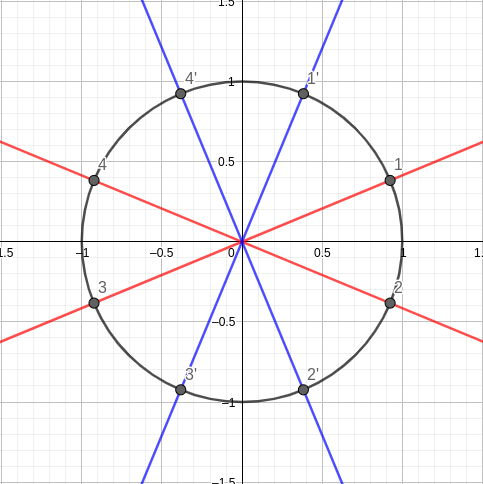시간 제한 메모리 제한 제출 정답 맞은 사람 정답 비율
4 초 512 MB 22 1 1 4.545%

## 문제

There was some complicated legend about navigation in futuristic space roads, and large hubs where they intersect, called cosmic crossroads. It was decided later on to skip this part and leave it up to your imagination, so let’s imagine how awesome it could have been, and proceed to the formal statement immediately.

Best regards from the problemsetter

Consider two sets of n lines passing through the point (0; 0; 0) in Euclidean three-dimensional space. Instead of actual lines, you are given two sets, 2n points in each set: ri = (xi; yi; zi) and rj′ = (xj′; yj′; zj′). These are the points where the lines intersect the unit sphere (two opposite points for each line).

It is known that the second set was obtained from the first one by some rotation around the origin and shuffling the order of the points. You have to find a permutation π1, . . . , π2n and the rotation φ of three-dimensional space such that, for all i,

rπi − φ(ri′)| ≤ 10−6.

The rotation φ should be described by point e = (x; y; z) and angle θ. It means we rotate the space around the axis from the origin to point e by angle θ following the right-hand rule (see notes).

It is guaranteed that the directions of the first set of lines were chosen uniformly at random, independently from each other.

## 입력

The first line of input contains a single integer n (2 ≤ n ≤ 4 · 104).

Each of the next 2n lines contains three real numbers xi, yi and zi describing points from the first set.

Each of the next 2n lines contains three real numbers xi′, yi′ and zi′ describing points from the second set.

All real numbers are given with the precision of up to 12 digits after decimal point.

It also holds that if point ri = (xi; yi; zi) lies in one of sets then the point −ri lies in that set as well.

It is guaranteed that solution exists and would still exist even with precision of 10−9 instead of 10−6.

## 출력

On the first line, print a single real number θ (−π ≤ θ ≤ π) describing the rotation angle.

On the second line, print three real numbers x, y, z (10−3 ≤ |x| + |y| + |z| ≤ 103) describing the point e on the rotation axis.

On the third line, print 2n integers π1, . . . , π2n.

Assume that rotation occurs in the sense prescribed by the right-hand rule (see notes).

## 예제 입력 1

2
0.923879533 0.382683432 0
0.923879533 -0.382683432 0
-0.923879533 -0.382683432 0
-0.923879533 0.382683432 0
0.382683432 0.923879533 0
0.382683432 -0.923879533 0
-0.382683432 -0.923879533 0
-0.382683432 0.923879533 0


## 예제 출력 1

-1.570796327
0.000000000 0.000000000 1.000000000
2 3 4 1


## 힌트

Just so you remember, rotation is done as follows:Example test case looks like this:The first set of points is {1, 2, 3, 4}, and the second set of points is {1′, 2′, 3′, 4′}. After the rotation of the second set of lines by −π/2 around axis (0; 0; 1), we obtain the following matching: {(1′; 2),(2′; 3),(3′; 4),(4′, 1)}.